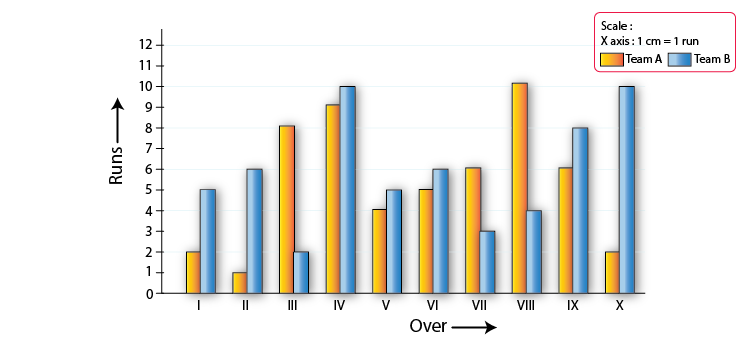# RD Sharma Solutions for Class 8 Maths Chapter 27 Introduction to Graphs Exercise 27.2

Exercise 27.2, mainly deals with the concept of construction of graphs. The exercise problems are well framed to give a better understanding of the concepts related to the construction of graphs. Students can make use of RD Sharma Class 8 Solutions to build a strong grip in solving the problems. On regular practice, students can achieve their goals of scoring high marks in their exams. Students can download the pdf of RD Sharma Class 8 Maths Solutions Exercise 27.2 Chapter 27 from the links available below.

## Download the Pdf of RD Sharma Solutions for Class 8 Maths Exercise 27.2 Chapter 27 Introduction to Graphs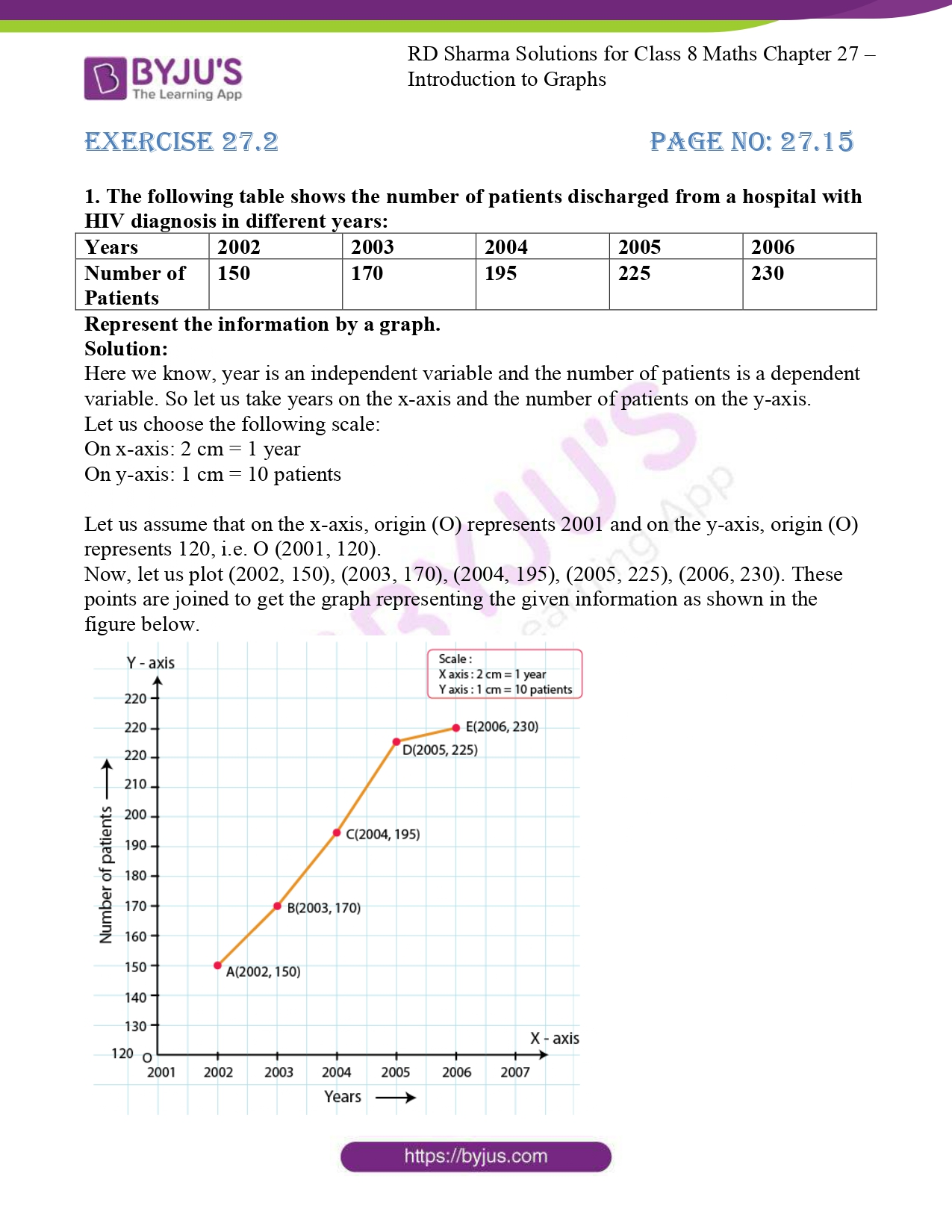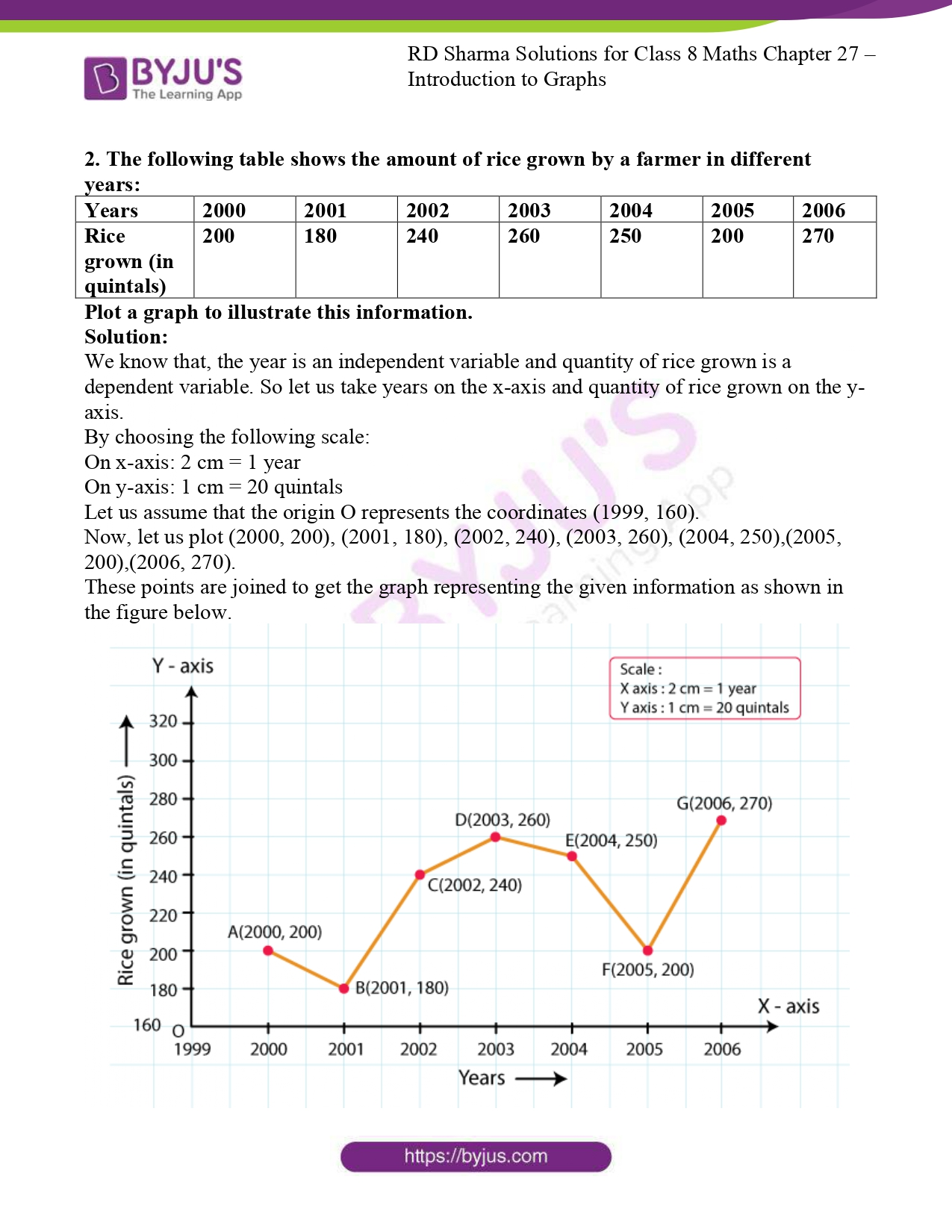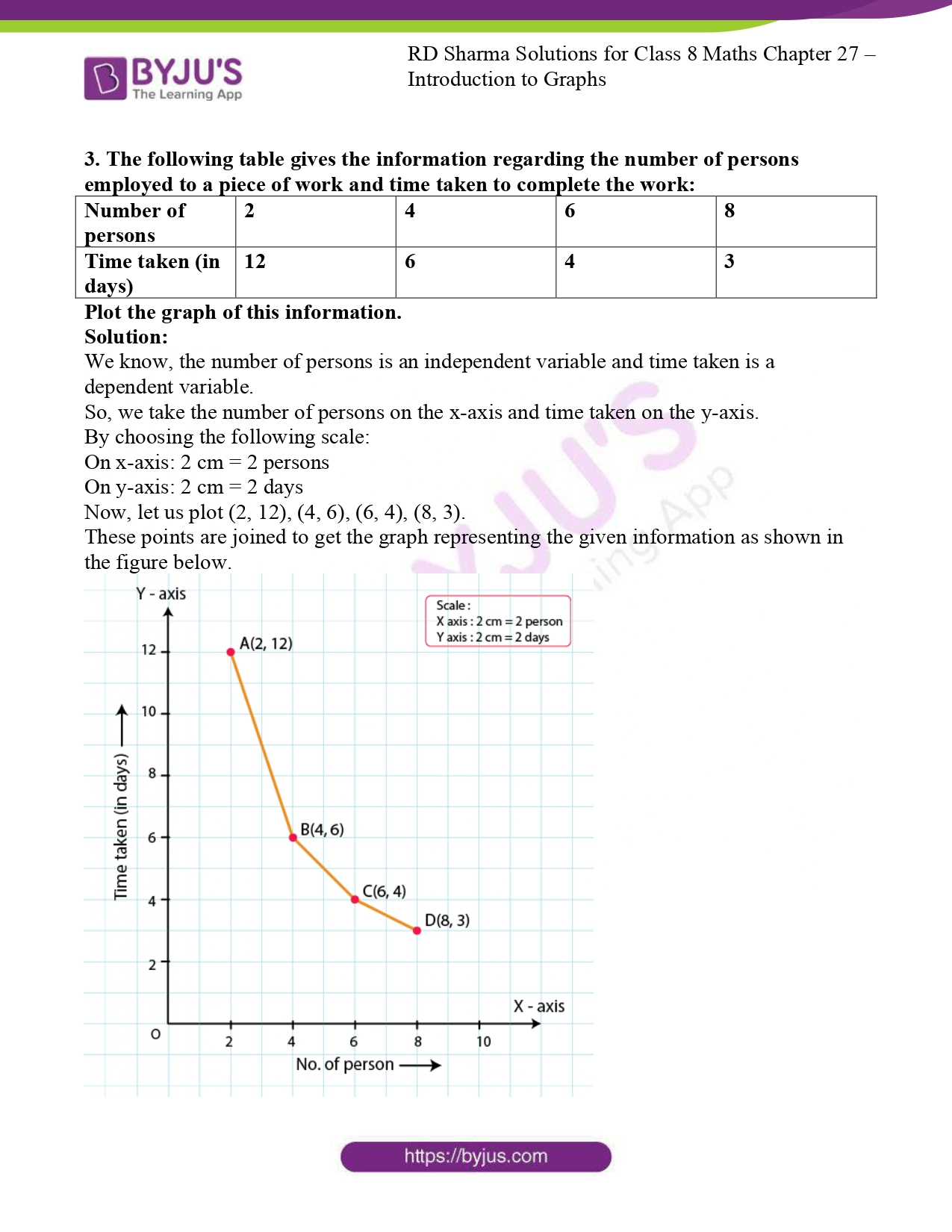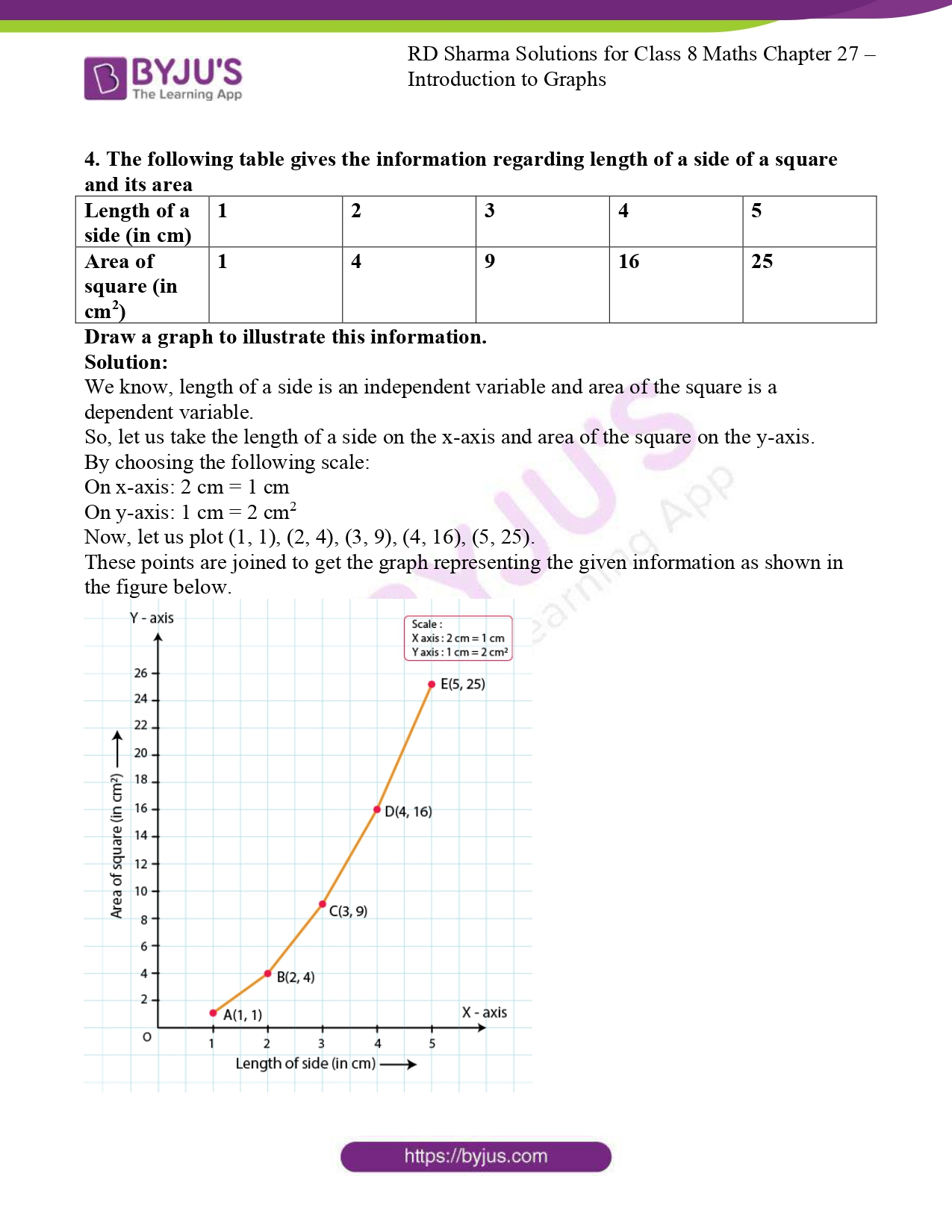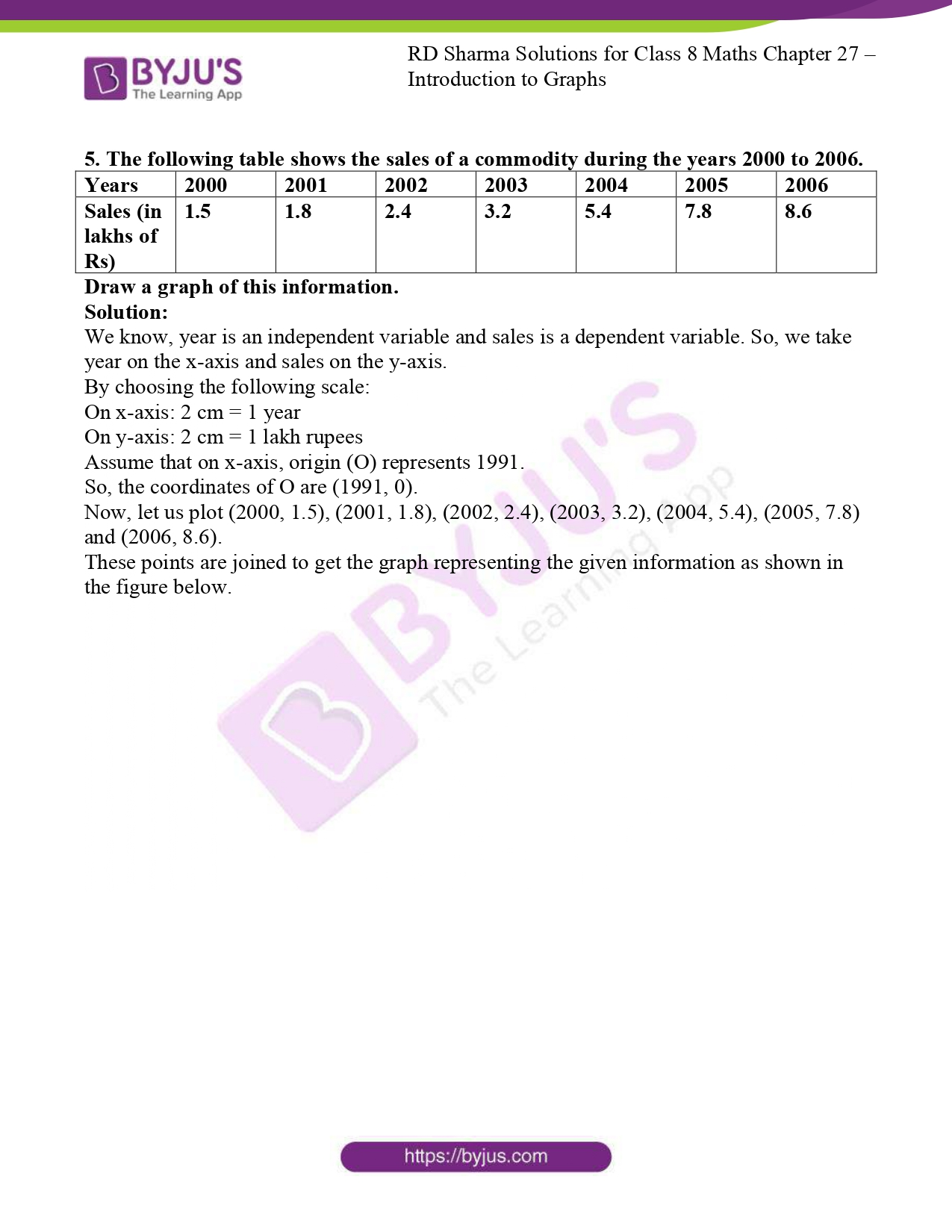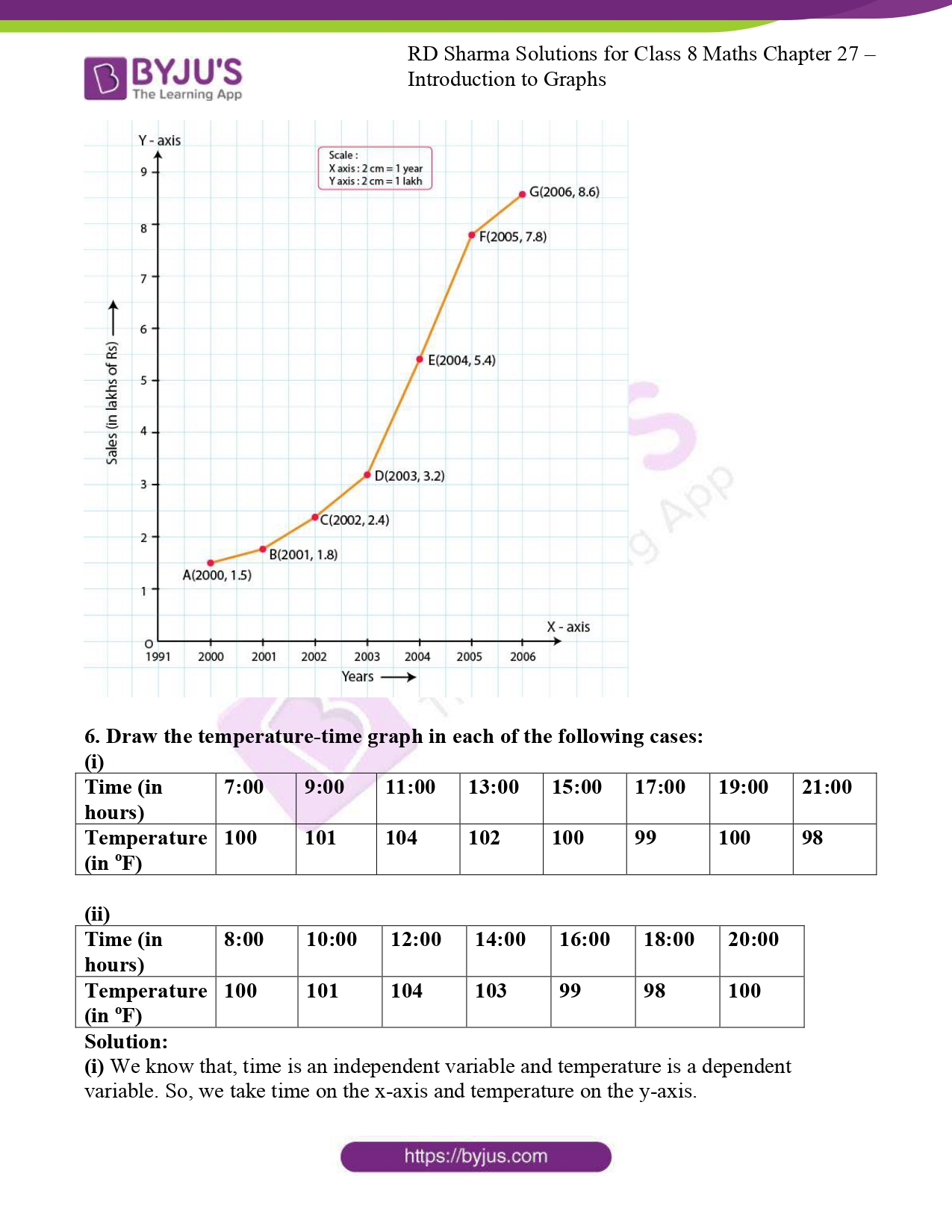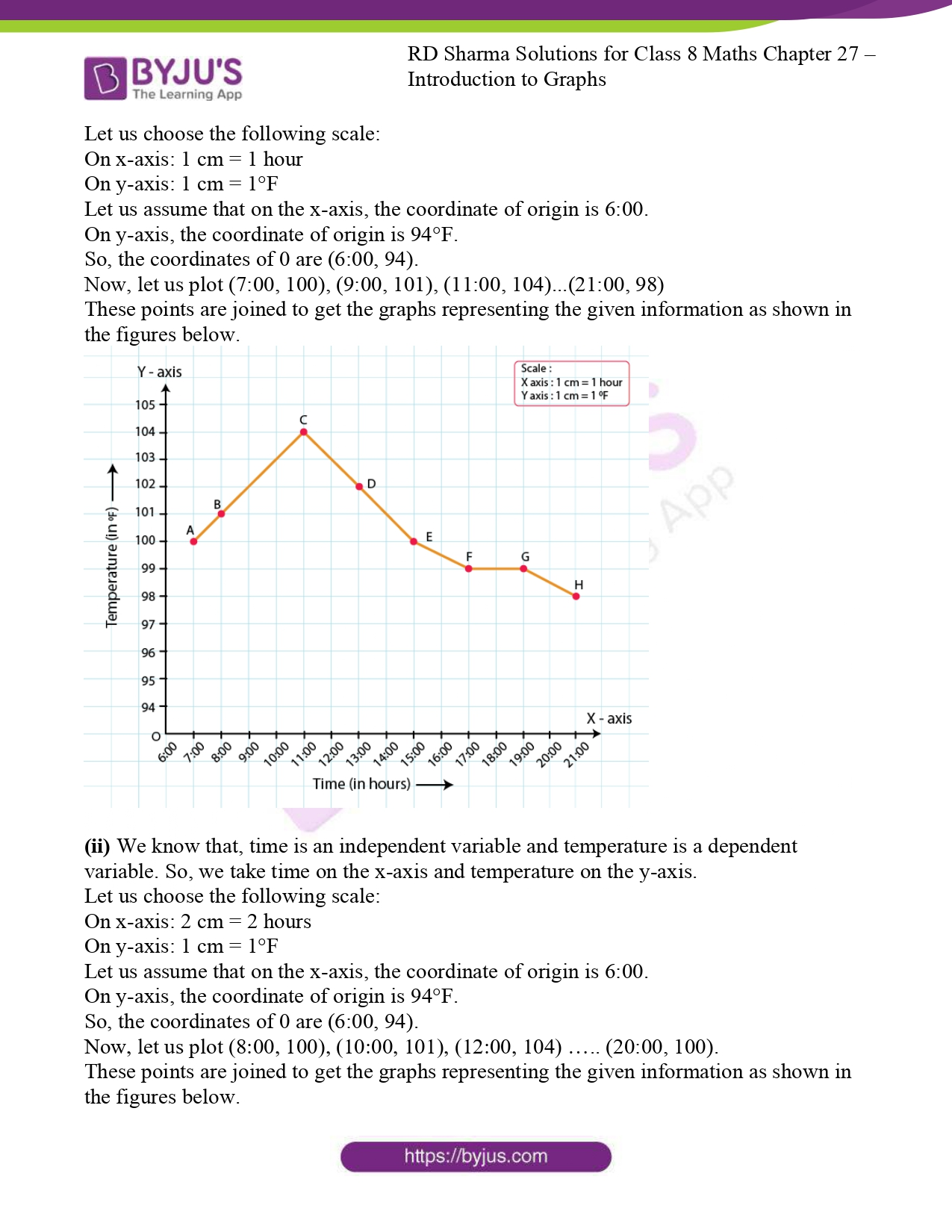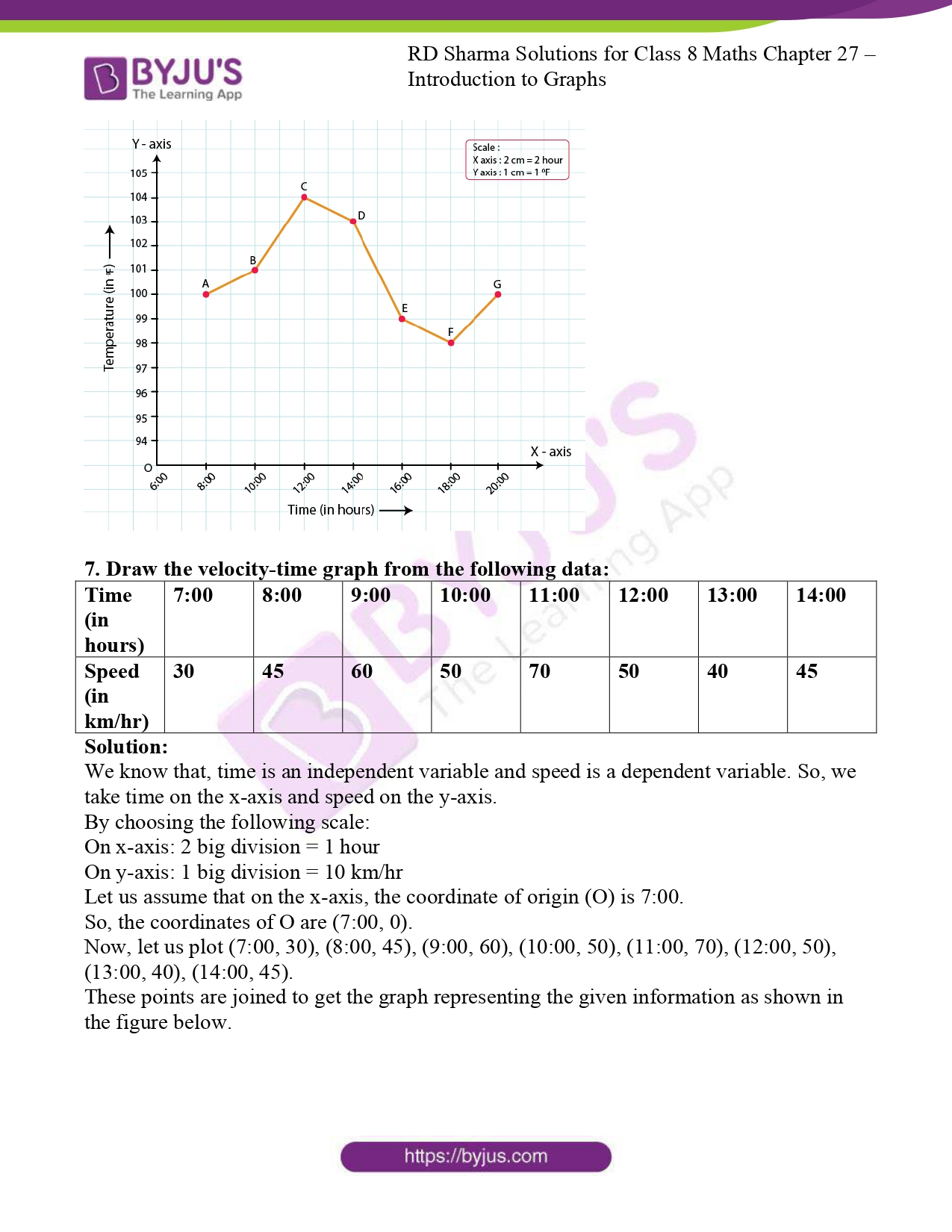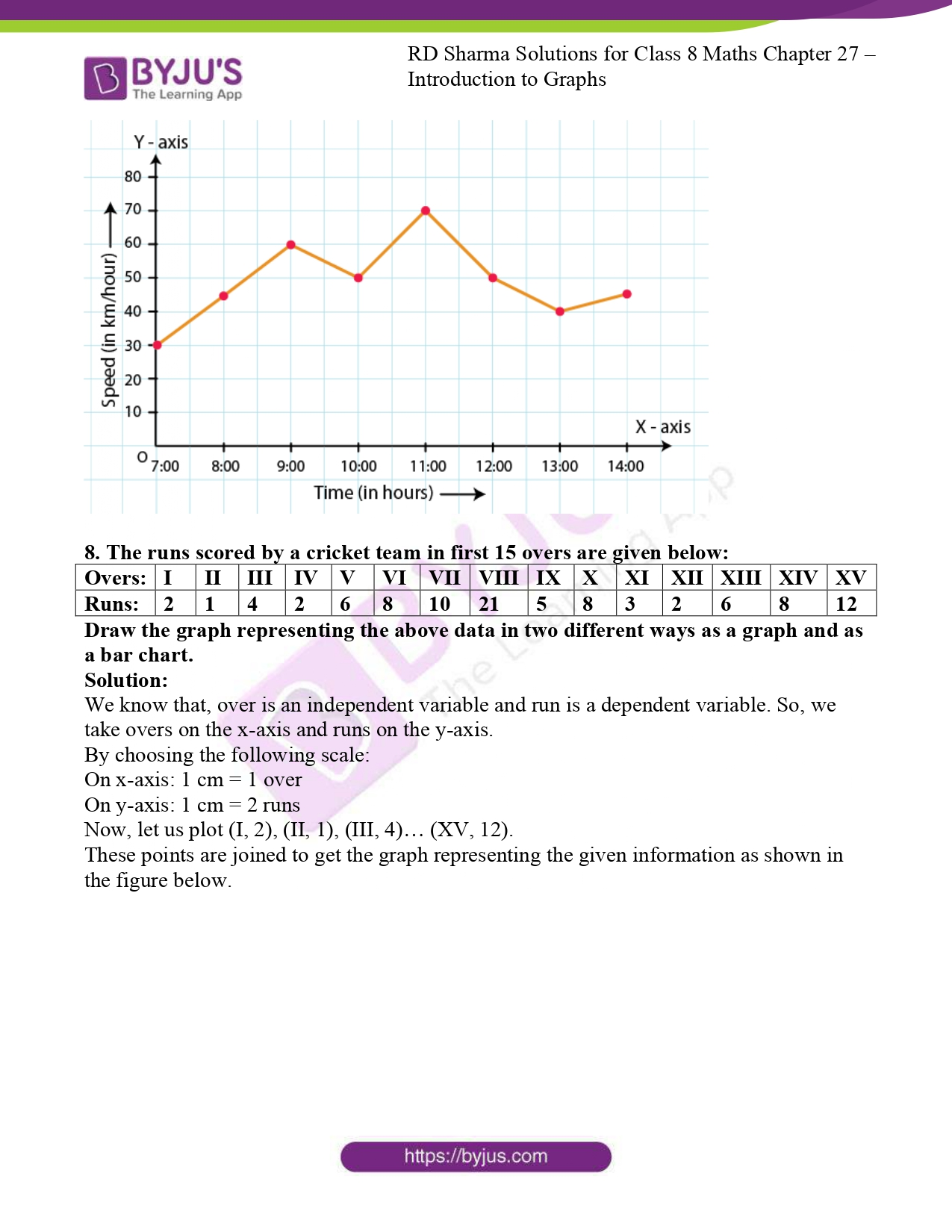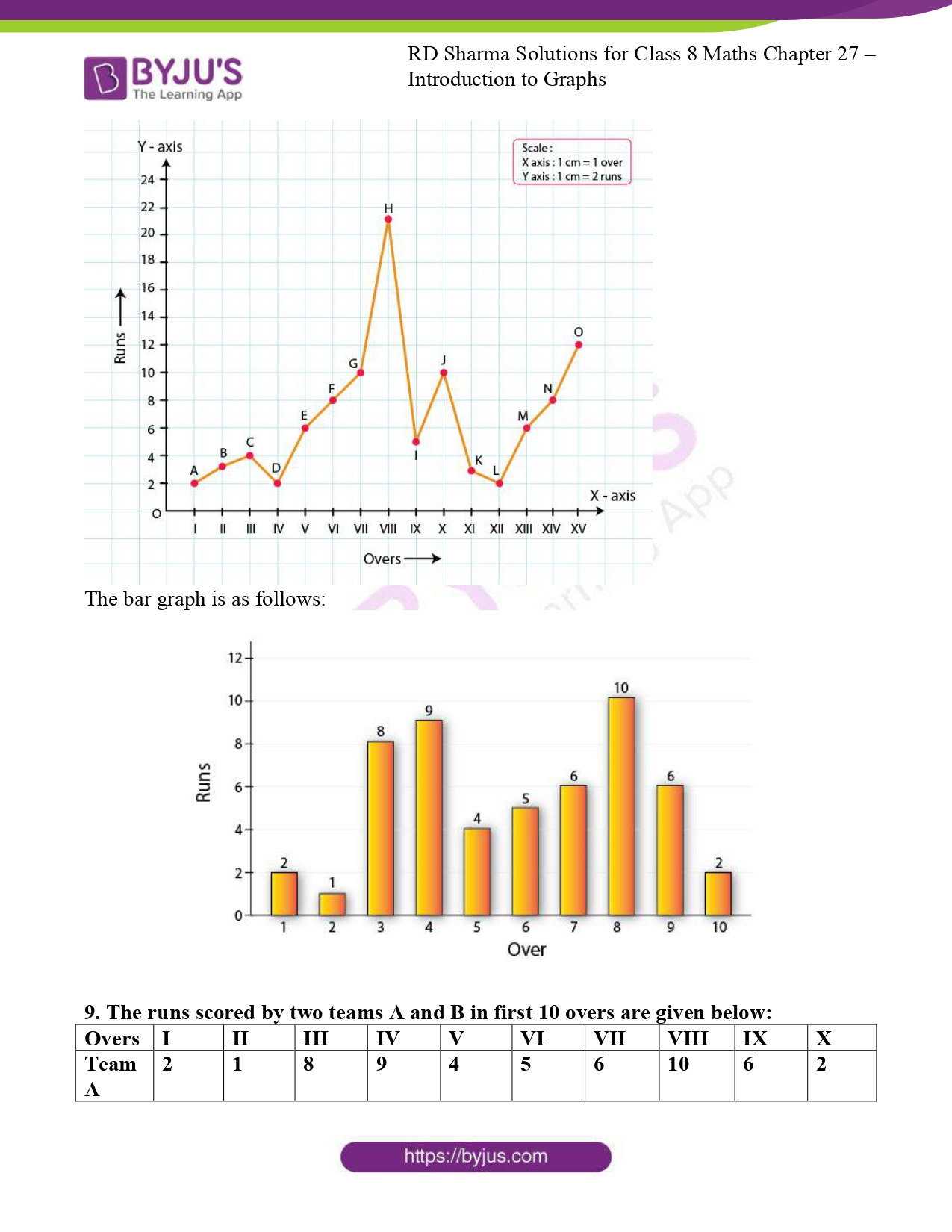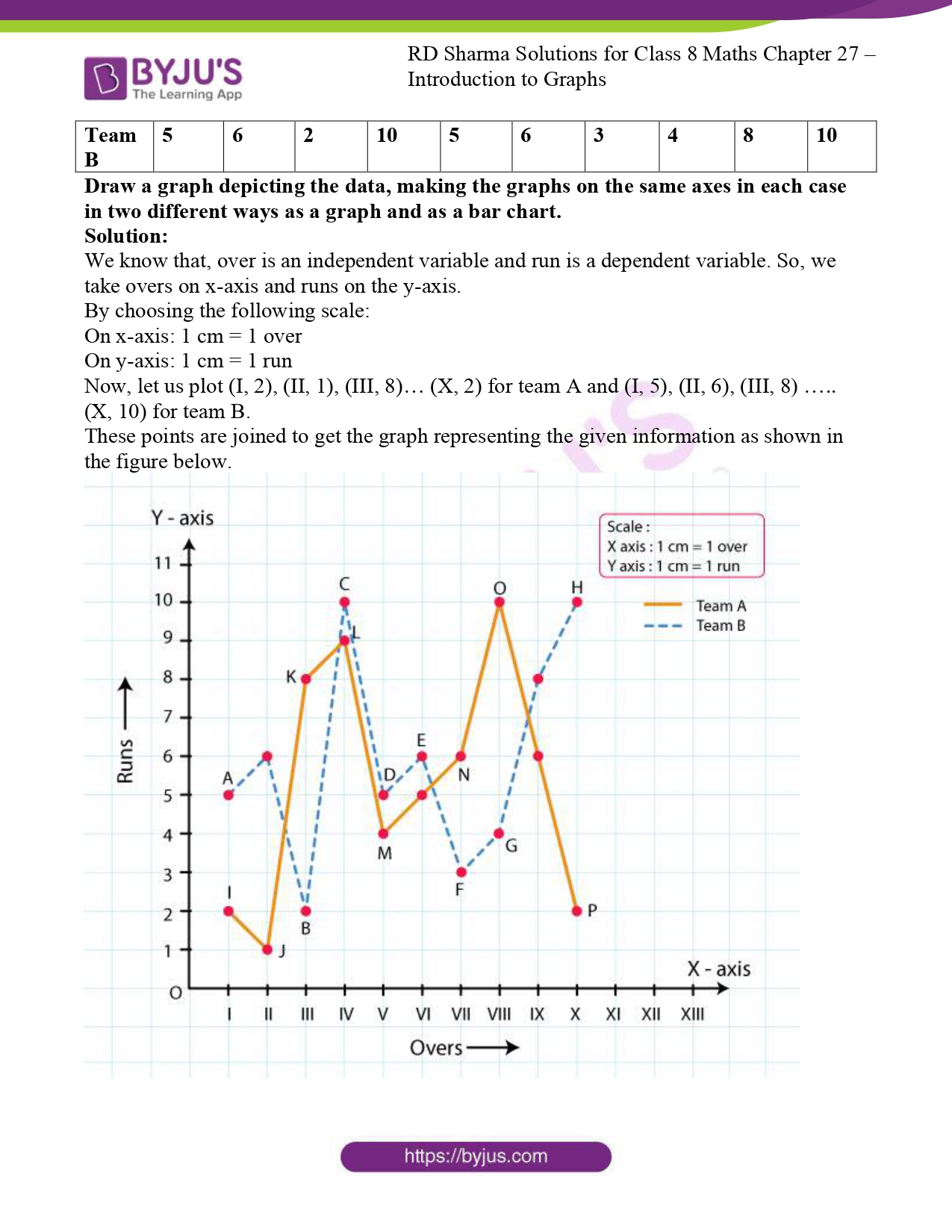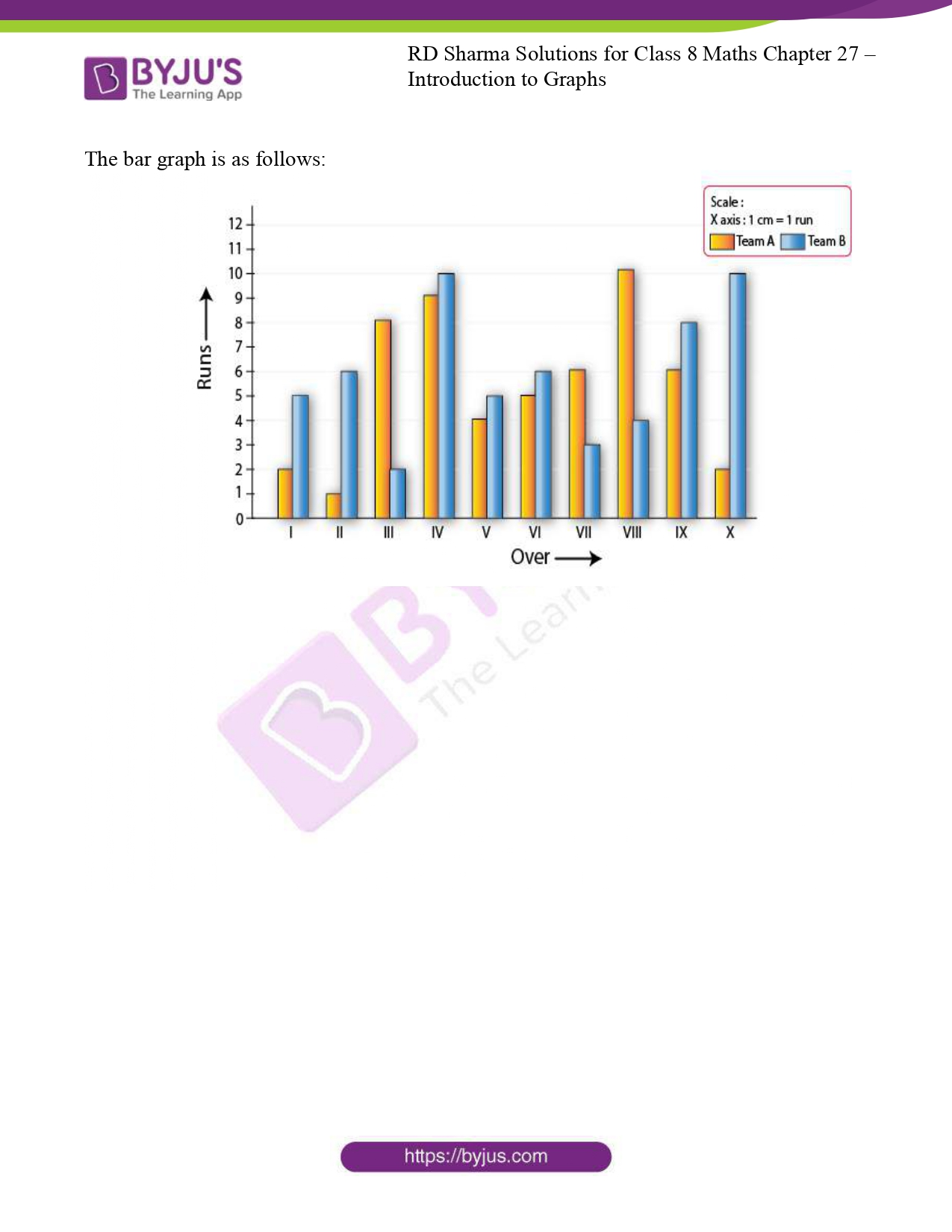### Access RD Sharma Solutions For Class 8 Maths Exercise 27.2 Chapter 27 Introduction to Graphs

1. The following table shows the number of patients discharged from a hospital with HIV diagnosis in different years:

 Years 2002 2003 2004 2005 2006 Number of Patients 150 170 195 225 230

Represent the information by a graph.

Solution:

Here we know, year is an independent variable and the number of patients is a dependent variable. So let us take years on the x-axis and the number of patients on the y-axis.

Let us choose the following scale:

On x-axis: 2 cm = 1 year

On y-axis: 1 cm = 10 patients

Let us assume that on the x-axis, origin (O) represents 2001 and on the y-axis, origin (O) represents 120, i.e. O (2001, 120).

Now, let us plot (2002, 150), (2003, 170), (2004, 195), (2005, 225), (2006, 230). These points are joined to get the graph representing the given information as shown in the figure below.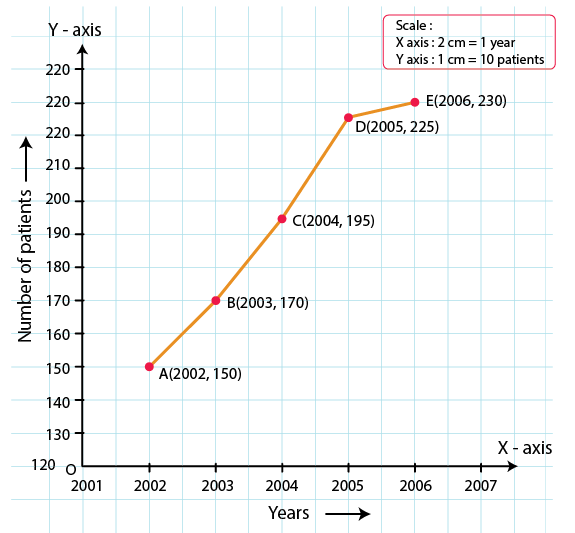2. The following table shows the amount of rice grown by a farmer in different years:

 Years 2000 2001 2002 2003 2004 2005 2006 Rice grown (in quintals) 200 180 240 260 250 200 270

Plot a graph to illustrate this information.

Solution:

We know that, the year is an independent variable and quantity of rice grown is a dependent variable. So let us take years on the x-axis and quantity of rice grown on the y-axis.

By choosing the following scale:

On x-axis: 2 cm = 1 year

On y-axis: 1 cm = 20 quintals

Let us assume that the origin O represents the coordinates (1999, 160).

Now, let us plot (2000, 200), (2001, 180), (2002, 240), (2003, 260), (2004, 250),(2005, 200),(2006, 270).

These points are joined to get the graph representing the given information as shown in the figure below.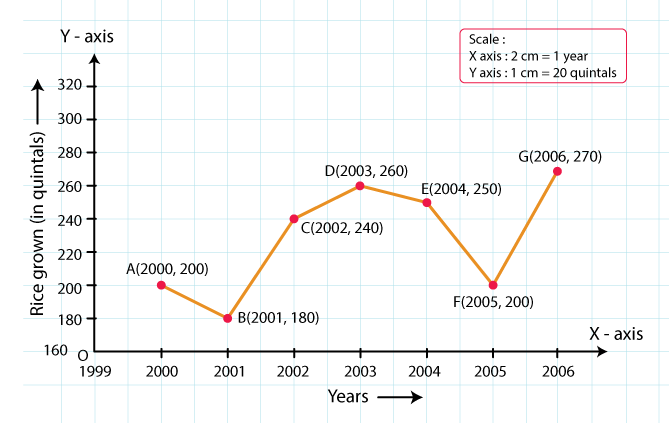3. The following table gives the information regarding the number of persons employed to a piece of work and time taken to complete the work:

 Number of persons 2 4 6 8 Time taken (in days) 12 6 4 3

Plot the graph of this information.

Solution:

We know, the number of persons is an independent variable and time taken is a dependent variable.

So, we take the number of persons on the x-axis and time taken on the y-axis.

By choosing the following scale:

On x-axis: 2 cm = 2 persons

On y-axis: 2 cm = 2 days

Now, let us plot (2, 12), (4, 6), (6, 4), (8, 3).

These points are joined to get the graph representing the given information as shown in the figure below.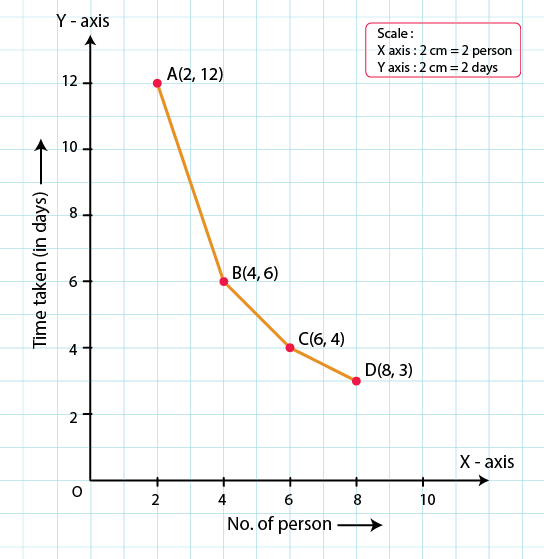4. The following table gives the information regarding length of a side of a square and its area

 Length of a side (in cm) 1 2 3 4 5 Area of square (in cm2) 1 4 9 16 25

Draw a graph to illustrate this information.

Solution:

We know, length of a side is an independent variable and area of the square is a dependent variable.

So, let us take the length of a side on the x-axis and area of the square on the y-axis.

By choosing the following scale:

On x-axis: 2 cm = 1 cm

On y-axis: 1 cm = 2 cm2

Now, let us plot (1, 1), (2, 4), (3, 9), (4, 16), (5, 25).

These points are joined to get the graph representing the given information as shown in the figure below.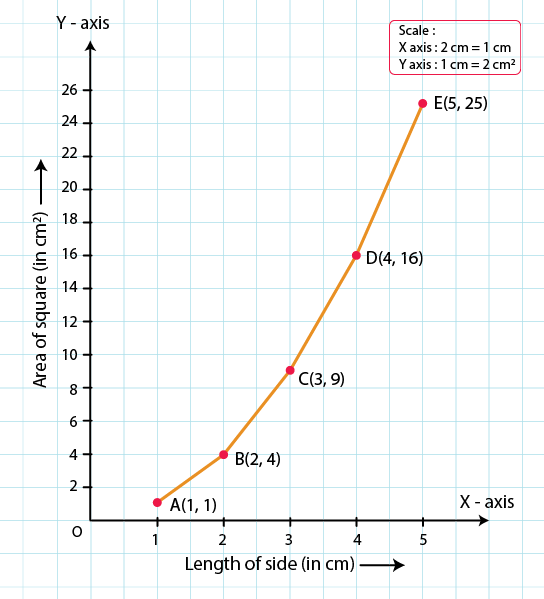5. The following table shows the sales of a commodity during the years 2000 to 2006.

 Years 2000 2001 2002 2003 2004 2005 2006 Sales (in lakhs of Rs) 1.5 1.8 2.4 3.2 5.4 7.8 8.6

Draw a graph of this information.

Solution:

We know, year is an independent variable and sales is a dependent variable. So, we take year on the x-axis and sales on the y-axis.

By choosing the following scale:

On x-axis: 2 cm = 1 year

On y-axis: 2 cm = 1 lakh rupees

Assume that on x-axis, origin (O) represents 1991.

So, the coordinates of O are (1991, 0).

Now, let us plot (2000, 1.5), (2001, 1.8), (2002, 2.4), (2003, 3.2), (2004, 5.4), (2005, 7.8) and (2006, 8.6).

These points are joined to get the graph representing the given information as shown in the figure below.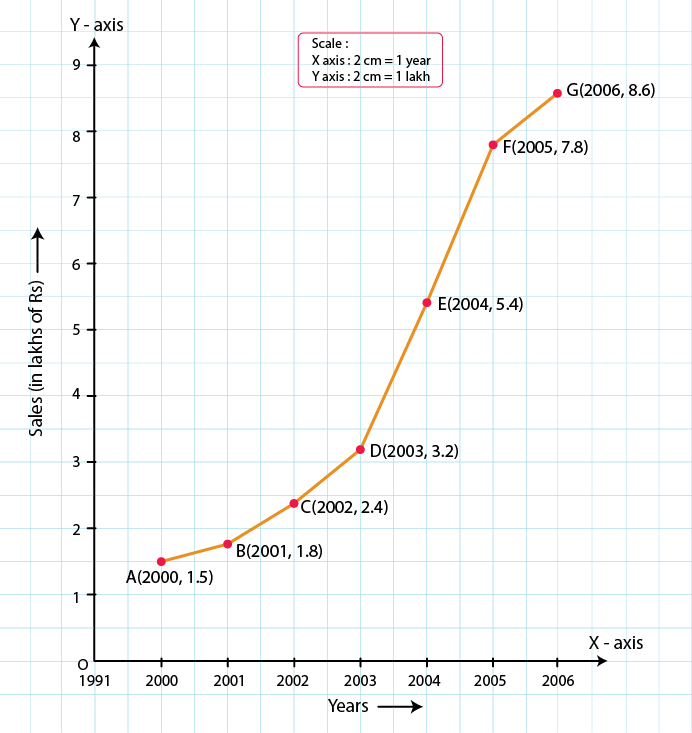6. Draw the temperature-time graph in each of the following cases:

(i)

 Time (in hours) 7:00 9:00 11:00 13:00 15:00 17:00 19:00 21:00 Temperature (in oF) 100 101 104 102 100 99 100 98

(ii)

 Time (in hours) 8:00 10:00 12:00 14:00 16:00 18:00 20:00 Temperature (in oF) 100 101 104 103 99 98 100

Solution:

(i) We know that, time is an independent variable and temperature is a dependent variable. So, we take time on the x-axis and temperature on the y-axis.

Let us choose the following scale:

On x-axis: 1 cm = 1 hour

On y-axis: 1 cm = 1°F

Let us assume that on the x-axis, the coordinate of origin is 6:00.

On y-axis, the coordinate of origin is 94°F.

So, the coordinates of 0 are (6:00, 94).

Now, let us plot (7:00, 100), (9:00, 101), (11:00, 104)…(21:00, 98)

These points are joined to get the graphs representing the given information as shown in the figures below.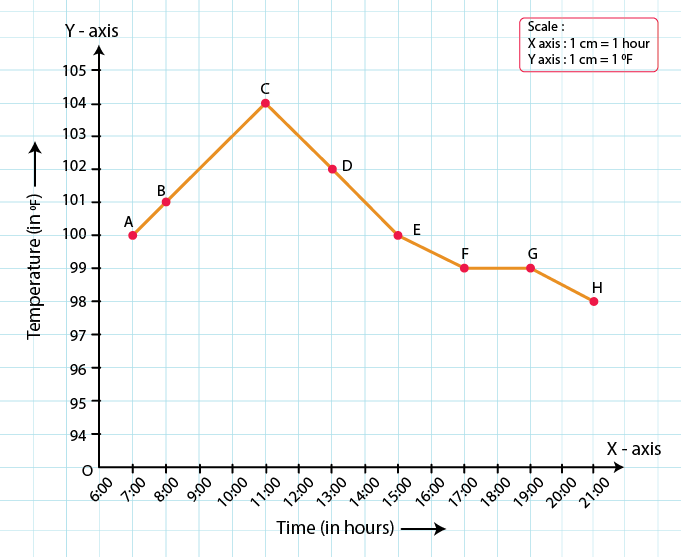(ii) We know that, time is an independent variable and temperature is a dependent variable. So, we take time on the x-axis and temperature on the y-axis.

Let us choose the following scale:

On x-axis: 2 cm = 2 hours

On y-axis: 1 cm = 1°F

Let us assume that on the x-axis, the coordinate of origin is 6:00.

On y-axis, the coordinate of origin is 94°F.

So, the coordinates of 0 are (6:00, 94).

Now, let us plot (8:00, 100), (10:00, 101), (12:00, 104) ….. (20:00, 100).

These points are joined to get the graphs representing the given information as shown in the figures below.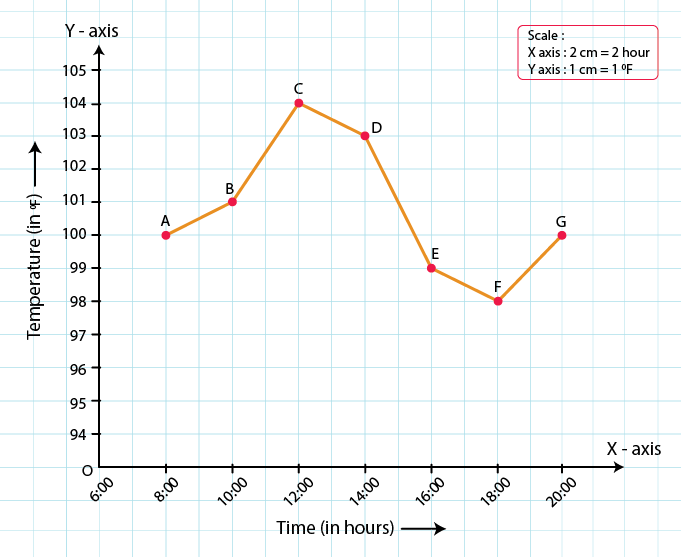7. Draw the velocity-time graph from the following data:

 Time (in hours) 7:00 8:00 9:00 10:00 11:00 12:00 13:00 14:00 Speed (in km/hr) 30 45 60 50 70 50 40 45

Solution:

We know that, time is an independent variable and speed is a dependent variable. So, we take time on the x-axis and speed on the y-axis.

By choosing the following scale:

On x-axis: 2 big division = 1 hour

On y-axis: 1 big division = 10 km/hr

Let us assume that on the x-axis, the coordinate of origin (O) is 7:00.

So, the coordinates of O are (7:00, 0).

Now, let us plot (7:00, 30), (8:00, 45), (9:00, 60), (10:00, 50), (11:00, 70), (12:00, 50), (13:00, 40), (14:00, 45).

These points are joined to get the graph representing the given information as shown in the figure below.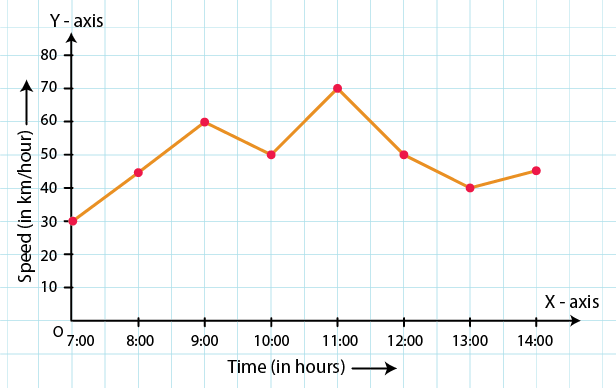8. The runs scored by a cricket team in first 15 overs are given below:

 Overs: I II III IV V VI VII VIII IX X XI XII XIII XIV XV Runs: 2 1 4 2 6 8 10 21 5 8 3 2 6 8 12

Draw the graph representing the above data in two different ways as a graph and as a bar chart.

Solution:

We know that, over is an independent variable and run is a dependent variable. So, we take overs on the x-axis and runs on the y-axis.

By choosing the following scale:

On x-axis: 1 cm = 1 over

On y-axis: 1 cm = 2 runs

Now, let us plot (I, 2), (II, 1), (III, 4)… (XV, 12).

These points are joined to get the graph representing the given information as shown in the figure below.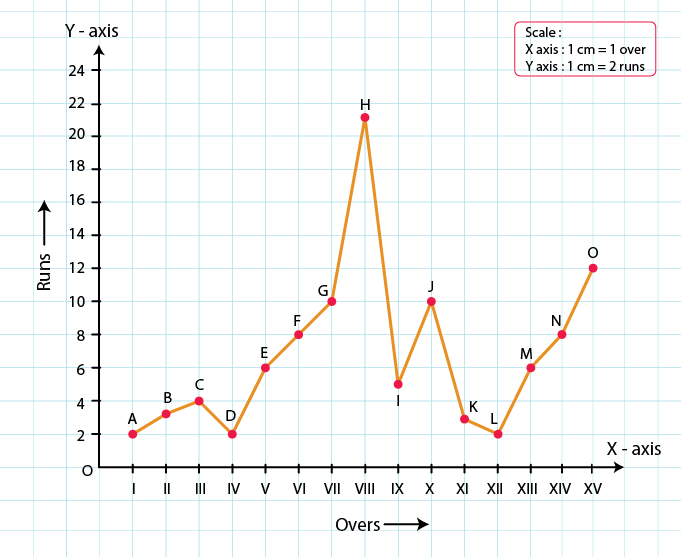The bar graph is as follows: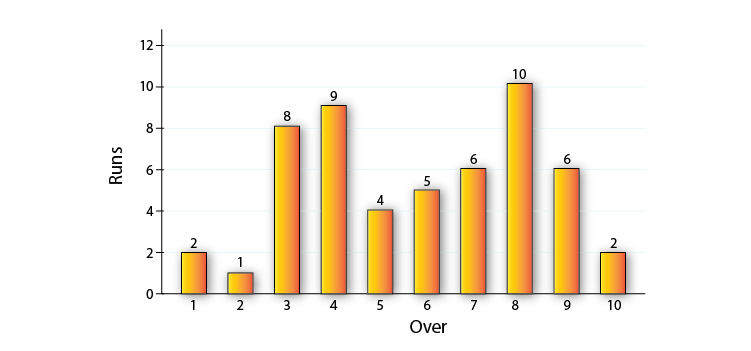9. The runs scored by two teams A and B in first 10 overs are given below:

 Overs I II III IV V VI VII VIII IX X Team A 2 1 8 9 4 5 6 10 6 2 Team B 5 6 2 10 5 6 3 4 8 10

Draw a graph depicting the data, making the graphs on the same axes in each case in two different ways as a graph and as a bar chart.

Solution:

We know that, over is an independent variable and run is a dependent variable. So, we take overs on x-axis and runs on the y-axis.

By choosing the following scale:

On x-axis: 1 cm = 1 over

On y-axis: 1 cm = 1 run

Now, let us plot (I, 2), (II, 1), (III, 8)… (X, 2) for team A and (I, 5), (II, 6), (III, 8) ….. (X, 10) for team B.

These points are joined to get the graph representing the given information as shown in the figure below.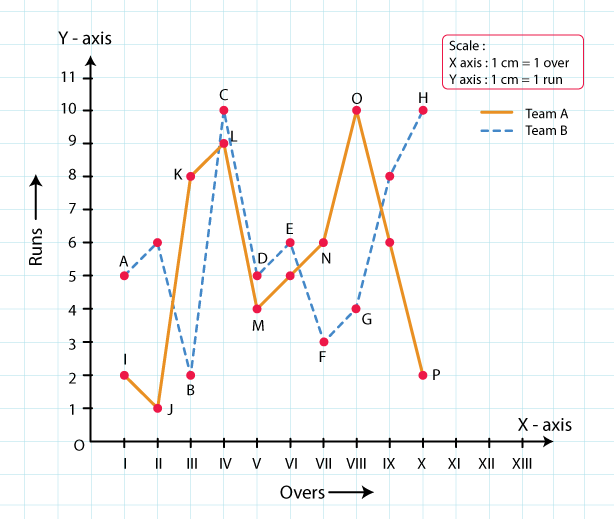The bar graph is as follows: